# Chapter 1 : Introduction to Polynomial

### Topics covered in this snack-sized chapter:

#### Polynomials arrow_upward

• Polynomials are mathematical expressions consisting of a sum of variables and exponents.

• ###### Example:

• 2x2 + 4x + 3
• Polynomials DO NOT contain:
• Square roots of variables
• Negative exponents
• Fractional powers
• No variables in the denominator of any fraction

#### General Form arrow_upward

• Let n be a non-negative integer.
• A polynomial function of degree n in the variable x is a function defined by:
•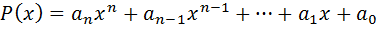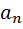is called the leading coefficient.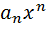is called the dominating term.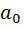is called the constant term.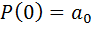is the y-intercept of the graph of.

#### Polynomials: Parts arrow_upward

P(x) = 5x3 + 3x + 7

• 5 is the leading coefficient.
• 5x3 is the dominating term.
• The degree of this polynomial is 3 (Exponent of the leading term).
• 7 is the constant.
• P(0) = 7 is the y-intercept of the graph of P.

• #### Polynomial Graphs arrow_upward

• Polynomial graphs are smooth and continuous.
• The graph shown below represents a polynomial function f(x) = x2 .
•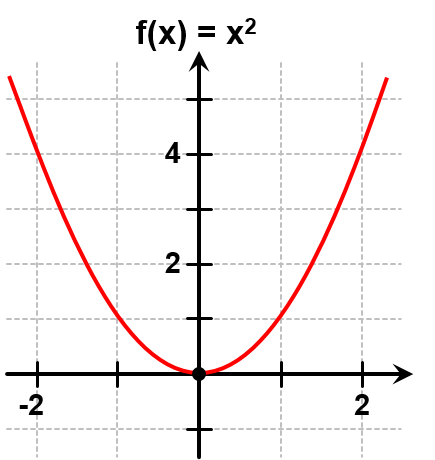Type of Graph Properties Smooth Graph Rounded curves with no sharp corners. Continuous Graph Graph has no breaks and can be drawn without lifting the pencil from the rectangular coordinate system.

#### Like and Unlike Terms arrow_upward

 Like Terms Unlike terms The like terms have same variable and same degree. The unlike terms have different variables and different degrees. 2x and 4x are the like terms. 3x2 and 4y3 are the unlike terms.

#### Degree of Polynomials arrow_upward

• The degree of a polynomial is the largest exponent in the polynomial.
• When the variable does not have an exponent, it is assumed to be 1.
• Examples:
• 3x is the first degree polynomial.
• 3x2 is the second degree polynomial. 3x5 - 4x + 3 is the fifth degree polynomial.

#### Coefficient arrow_upward

• The coefficient is the constant term placed before a variable.

• ###### Example 1:

• In terms 3x and ax, 3 and a are coefficients of x.

• ###### Example 2:

• Determine coefficient of each term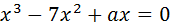?
• Solution:

•  Term Coefficient x3 1 -7x2 -7 ax a

#### Polynomial Classification arrow_upward

 Type Examples Monomial: Has one term 5y or – 8x Binomial: Has two terms -3x2 + 2 or 9y – 2y2 Trinomial: Has three terms -3x2 + 3x + 2 or 9y3 – 2y2 + y

Classification of Polynomials Based on Degree:

 Degree Name Example - Zero 0 0 (Nonzero) constant 1 1 Linear a + 1 2 Quadratic a2 + 1 3 Cubic a3 + 1 4 Quartic(or biquadratic) a4 + 1 5 Quintic a5 + 1 6 Hexic a6 + 1 7 Septic a7 + 1 8 Octic a8 + 1 9 Nonic a9 + 1 10 Decic a10 + 1

#### Polynomials in One Variable arrow_upward

• Algebraic expressions that consist of terms in the form of axn .
• n is a non-negative integer.
• x is a real number.
• a is called the coefficient of a term.
• Example 1: 4x.
• Here we have one term with variable x and the exponent (or degree) as 1.
• Example 2: 3x2 + 2.
• Here we have two terms, with one variable x having the exponent (or degree) as 2 and another is constant value 2.

#### Degree of a Polynomial with One Variable arrow_upward

• Example 1: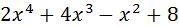• This is a polynomial with one variable.
• The highest degree is 4.
• Example 2: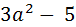• This is a polynomial with one variable.
• The highest degree is 2.

#### Polynomials in Two Variable arrow_upward

• Polynomials in two variables are algebraic expressions consisting of terms in the form:
•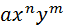• The degree of the polynomial is the largest sum of the exponents of two variables.

• ###### Example:

•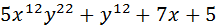• Degree of the above polynomial is
•     12 + 22 = 34

• Place like terms together.

• ###### Example:

• Solve (5x + 7y) + (2x - y)

• ###### Solution:

(5x + 7y) + (2x - y)

• Group like terms together:
•      (5x + 2x) + (7y - y)

• On simplifying the terms, we get:
•     = (7x + 6y)

#### Subtracting Polynomials arrow_upward

• To subtract polynomials, first reverse the sign of each term you are subtracting.
• In other words turn "+" into "-", and "-" into "+" and then add as usual.

• ###### Example:

• Solve (4x2 - 4) - (-x2 - 4x + 4)

• ###### Solution:

• First arrange the terms:
•       4x2 – 4 + x2 + 4x - 4
•    = 4x2 + x2 + 4x – 4 – 4
•    = 5x2 + 4x – 8

#### Multiplying Polynomials arrow_upward

• To multiply two polynomials:
• Multiply each term in one polynomial by each term in the other polynomial.
• Add the resulting terms together, and simplify if needed.

###### Example:

• (x + 3)(x) + (x + 3)(2)

• ###### Solution:

• First arrange the terms as:
•     = (x + 3)(x) + (x + 3)(2)

= x(x) + 3(x) + x(2) + 3(2)

= x2 + 3x + 2x + 6

= x2 + 5x + 6

#### Polynomial Division Algorithm arrow_upward

• If f(x) and d(x) are polynomials,
• With d(x) = divisor and f(x) = dividend
• And the degree of d(x) is less than or equal to the degree of f(x).
• Then there exist unique polynomials q(x) and r(x) such that:#### Steps for Polynomial Division arrow_upward

• Arrange the terms of both the dividend and the divisor in descending powers of any variable.
• Divide the first term in the dividend by the first term in the divisor.
• The result is the first term of the quotient.
• Multiply every term in the divisor by the first term in the quotient.
• Write the resulting product beneath the dividend with like terms lined up.
• Subtract the product from the dividend.
• Bring down the next term in the original dividend and write it next to the remainder to form a new dividend.
• Use this new expression as the dividend and repeat this process until the remainder can no longer be divided.
• This will occur when the degree of the remainder (the highest exponent on a variable in the remainder) is less than the degree of the divisor.

• #### Long Division Method arrow_upward

• Divide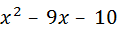by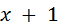.
• First, set up the division.
• Ignore the other terms and look just at the leading x of the divisor and the leading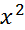of the dividend.
•• Divideby the leading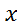.
• Put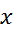on top.
•• Take that x, and multiply it through the divisor, x + 1.
• Multiply the x (on top) by the x (on the “side”), and carry the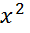underneath.• Then multiply the x (on top) by the 1 (on the “side”), and carry the 1x underneath.• To subtract the polynomials, change all the signs in the second line and then add down.• The first term (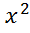) will cancel out,• Now, carry down that last term -10,• Now look at the x from the divisor and the new leading term, –10x, in the bottom line of the division.
• Divide the –10x by the x, it ends up with –10, so put that on top:• Now multiply –10 by the leading x:• Now multiply the –10 (on top) by the 1 (on the “side”), and carry the –10 to the bottom:• Change the signs of all the terms in the bottom row (to subtract).
••• The solution to this division is: x - 10

• ###### Proof: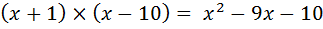L.H.S. =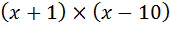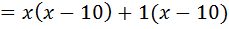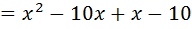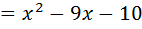= R.H.S.

Hence proved L.H.S = R.H.S

#### Thank You from Kimavi arrow_upward

• Please email us at Admin@Kimavi.com and help us improve this tutorial.